# Morse index theorem

(diff) ← Older revision | Latest revision (diff) | Newer revision → (diff)

## Statement

### Setup

Let$\R^n$ be Euclidean space, and let$G$ be the linear space of piecewise$C^\infty$-maps from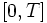$[0,T]$ to$\R^n$. Let$H$ denote the subspace of$G$ comprising maps which are zero at the endpoints (viz maps$u$ satisfying$u(0) = u(T) = 0$).

Let$P$ be a$C^\infty$-map from$[0,T]$ into the collection of self-adjoint linear transformations on$\R^n$.

Let$U$ be the unique$C^\infty$-map from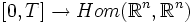$[0,T]\to Hom(\R^n,\R^n)$ such that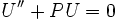$U'' + PU = 0$.

Define:

• The multiplicity of$t \in [0,T]$ is the nullity of$U(t)$
•$t \in [0,T]$ is said to be a focal point if its multiplicity is positive

Define the index form corresponding to$P$ as the following bilinear form on$H$: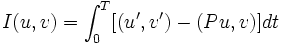$I(u,v) = \int_0^T [(u',v') - (Pu,v)] dt$

### Statement part

• The index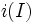$i(I)$ (viz the index of the index form) is the sum of multiplicities of all focal points in the open interval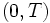$(0,T)$
• The nullity$n(I)$ (viz the nullity of the index form) is the multiplicity of$T$.